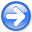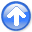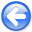Fast log-decon with a quasi-Newton solver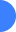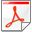Next: The limited-memory BFGS method Up: Guitton: Quasi-Newton log-decon Previous: CONCLUSION

# APPENDIX

The L-BFGS method is suitable for smooth functions where local minima exist. It is not a method for global optimization where the global minimum is sought. L-BFGS is presented here in general terms using global definitions for the different variables: it does not follow the notations of the log-decon method.

We define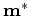a local minimizer for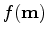and we assume thatandsatisfy the standard requirements'':

1.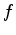is twice differentiable,
2.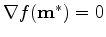,
3.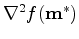is positive definite , i.e.,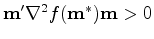for all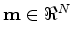(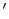denotes the adjoint).
where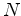is the dimension of the model vector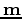and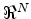the real space for the model vector. Any vectorthat satisfies the standard requirements is a local minimizer of.

Newton's method is an iterative process where the solution to the problem is updated as follows: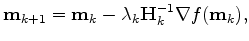(1)

where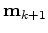is the updated solution at iteration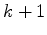,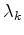the step length computed by a line search that ensures a sufficient decrease ofandthe Hessian (or second derivative). In many circumstances the inverse of the Hessian can't be computed directly. It happens for example when the matrix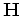is too big or when operators are used rather than matrices. Fortunately we might be able to compute an approximation of the Hessian of. This strategy gives birth to quasi-Newton methods where the way in which the Hessian is computed determines the method (Kelley, 1999).

A possible update of the Hessian is given by the BFGS technique Fletcher (1970); Goldfarb (1970); Shanno (1970); Broyden (1969). The BFGS update is given by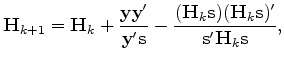(2)

whereand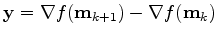. In practice, however, we rather write the previous equation in terms of the inverse matrices. We have then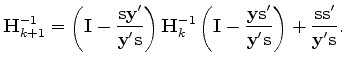(3)

In addition, we use the history of the iterations to compute the new Hessian rather than a full storage of the matrix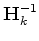. This requires that a gradient step vector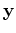and a solution step vectorare kept in memory after each iteration. Consequently this method might not been affordable with large data and model space. In the next section a modified version of the BFGS method that limits the storage needed to compute the update of the Hessian is proposed.

SubsectionsFast log-decon with a quasi-Newton solverNext: The limited-memory BFGS method Up: Guitton: Quasi-Newton log-decon Previous: CONCLUSION

2012-10-29